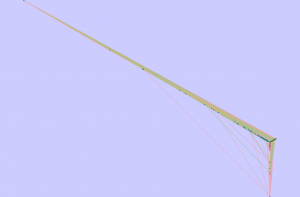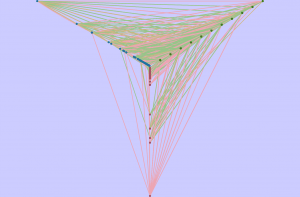[This article was first published on Deciphering life: One bit at a time :: R, and kindly contributed to R-bloggers]. (You can report issue about the content on this page here)
Want to share your content on R-bloggers? click here if you have a blog, or here if you don't.

I found out about HivePlots this past summer, and although I thought they looked incredibly useful and awesome, I didn't have a personal use for them at the time, and therefore put off doing anything with them. That recently changed when I encountered some particularly nasty hairballs of force-directed graphs. Unfortunately, the HiveR package does not create interactive hiveplots (at least for 2D), and that is particularly important for me. I don't necessarily want to be able to compare networks (one of the selling points made by Martin Krzywinski), but I do want to be able to explore the networks that I create. For that reason I have been a big fan of the RCytoscape Bioconductor package since I encountered it, as it allows me to easily create graphs in R, and then interactively and programmatically explore them in Cytoscape

So I decided last week to see how hard it would be to generate a hive plot that could be visualized and interacted with in Cytoscape. For this example I'm going to use the data in the HiveR package, and actually use the structures already encoded, because they are useful.

require(RCytoscape)
require(HiveR)
require(graph)
options(stringsAsFactors = F)

dataDir <- file.path(system.file("extdata", package = "HiveR"), "E_coli")
EC1 <- dot2HPD(file = file.path(dataDir, "E_coli_TF.dot"), node.inst = NULL,
edge.inst = file.path(dataDir, "EdgeInst_TF.csv"), desc = "E coli gene regulatory network (RegulonDB)",
axis.cols = rep("grey", 3))

## No node instructions provided, proceeding without them

str(EC1)

## List of 5
##  $nodes :'data.frame': 1597 obs. of 6 variables: ## ..$ id    : int [1:1597] 1 2 3 4 5 6 7 8 9 10 ...
##   ..$lab : chr [1:1597] "pstB" "hybE" "fadE" "phnF" ... ## ..$ axis  : int [1:1597] 1 1 1 1 1 1 1 1 1 1 ...
##   ..$radius: num [1:1597] 1 1 1 1 1 1 1 1 1 1 ... ## ..$ size  : num [1:1597] 1 1 1 1 1 1 1 1 1 1 ...
##   ..$color : chr [1:1597] "transparent" "transparent" "transparent" "transparent" ... ##$ edges    :'data.frame':   3893 obs. of  4 variables:
##   ..$id1 : int [1:3893] 932 612 932 1510 1510 413 528 652 1396 400 ... ## ..$ id2   : int [1:3893] 832 620 51 525 797 151 5 1058 1396 1559 ...
##   ..$weight: num [1:3893] 1 1 1 1 1 1 1 1 1 1 ... ## ..$ color : chr [1:3893] "red" "red" "green" "green" ...
##  $desc : chr "E coli gene regulatory network (RegulonDB)" ##$ axis.cols: chr [1:3] "grey" "grey" "grey"
##  $type : chr "2D" ## - attr(*, "class")= chr "HivePlotData"  ## Process Data So here we have the data. The nodes is a data frame with the id, a label describing the node, which axis the node belongs on, and its radius, or how far out on the axis the node should be, as well as a size. These are all modifiable attributes that can be changed depending on how one wants to map different pieces of data. This of course is the beauty of hive plots, because they result in networks that are dependent on attributes that the user decides on. In this case, we have a transcription factor regulation network. I am going to point you to the previous links as to why a normal force-directed network diagram is not really that informative for these types of networks. I'm not out to convince you that HivePlots are useful, if you don't get it from the publication and examples, then you should stop here. This is more about how to do some calculations to lay them out and work with them in Cytoscape. Bryan has implemented some nice functions to work with this type of network and perform simple calculations to assign axes and locations based on properties of the nodes. For example, it is easy to locate nodes on an axis based on the total number of edges. EC2 <- mineHPD(EC1, option = "rad <- tot.edge.count") sumHPD(EC2) ## E coli gene regulatory network (RegulonDB) ## This hive plot data set contains 1597 nodes on 1 axes and 3893 edges. ## It is a 2D data set. ## ## Axis 1 has 1597 nodes spanning radii from 1 to 434 ## ## Axes 1 and 1 share 3893 edges  And then to assign the axis to be plotted on based on the whether edges are incoming (sink), outgoing (source), or both (manager). These are the types of decisions that influence whether you get anything insightful or useful out of a HivePlot, and changing these options can of course change the conclusions you will make on a particular network. EC3 <- mineHPD(EC2, option = "axis <- source.man.sink") sumHPD(EC3) ## E coli gene regulatory network (RegulonDB) ## This hive plot data set contains 1597 nodes on 3 axes and 3893 edges. ## It is a 2D data set. ## ## Axis 1 has 45 nodes spanning radii from 1 to 83 ## Axis 2 has 1416 nodes spanning radii from 1 to 11 ## Axis 3 has 136 nodes spanning radii from 2 to 434 ## ## Axes 1 and 2 share 400 edges ## Axes 1 and 3 share 21 edges ## Axes 3 and 2 share 3158 edges ## Axes 3 and 3 share 314 edges  We also remove any nodes that have zero edges. EC4 <- mineHPD(EC3, option = "remove zero edge") ## ## 125 edges that start and end on the same point were removed  And finally re-order the edges (not sure how this would affect plotting using Cytoscape). edges <- EC4$edges
edgesR <- subset(edges, color == "red")
edgesG <- subset(edges, color == "green")
edgesO <- subset(edges, color == "orange")
edges <- rbind(edgesO, edgesG, edgesR)
EC4$edges <- edges EC4$edges$weight = 0.5  ## Calculate Node Locations In this case we have three axes, so we are going to calculate the axes locations as 0, 120, and 240 degrees. However, we need to use radians, because the conversion from spherical to cartesian coordinates involves using cosine and sine, which in R is based on radians. r2xy <- function(inRad, inPhi) { x <- inRad * sin(inPhi) y <- inRad * cos(inPhi) cbind(x, y) } tmpDat <- EC4$nodes[, c("id", "axis", "radius")]
tmpDat$radius <- tmpDat$radius * 3  # bump it up as cytoscape coordinates are small
tmpDat$phi <- ((tmpDat$axis - 1) * 120) * (pi/180)

nodeXY <- r2xy(tmpDat$radius, tmpDat$phi)  # contains cartesian coordinates


## Create GraphNEL

Initialize the graph with the nodes and the edges.

hiveGraph <- new("graphNEL", nodes = as.character(EC4$nodes$id), edgemode = "directed")
hiveGraph <- addEdge(as.character(EC4$edges$id1), as.character(EC4$edges$id2),
hiveGraph)


We also want to put information we know about the nodes and edges in the graph, so that we can modify colors and stuff based on those attributes. For example, in this case we might want to modify the node color based on the axis it is on. Using attributes means we are not stuck using the colors that we previously assigned.

nodeDataDefaults(hiveGraph, "nodeType") <- ""

nodeTypes <- c(1 = "source", 2 = "man", 3 = "sink")
nodeData(hiveGraph, as.character(EC4$nodes$id), "nodeType") <- nodeTypes[as.character(EC4$nodes$axis)]

interactionType <- c(red = "repressor", green = "activator", orange = "dual")
edgeData(hiveGraph, as.character(EC4$edges$id1), as.character(EC4$edges$id2),
"interactionType") <- interactionType[EC4$edges$color]


## Transfer to Cytoscape

ccHive <- CytoscapeWindow("hiveTest", hiveGraph)
displayGraph(ccHive)

##  "nodeType"
##  "label"
##  "interactionType"


Now lets move those nodes to their positions based on the Hive Graph calculations.

setNodePosition(ccHive, as.character(EC4$nodes$id), nodeXY[, 1], nodeXY[, 2])
fitContent(ccHive)
setDefaultNodeSize(ccHive, 5)

##  TRUE


And set the colors based on attributes:

nodeColors <- hcl(h = c(0, 120, 240), c = 55, l = 45)  # darker for the nodes
edgeColors <- hcl(h = c(0, 120, 60), c = 45, l = 75)  # lighter for the edges

setNodeColorRule(ccHive, "nodeType", c("source", "man", "sink"), nodeColors,
"lookup")
setNodeBorderColorRule(ccHive, "nodeType", c("source", "man", "sink"), nodeColors,
"lookup")
setEdgeColorRule(ccHive, "interactionType", c("repressor", "activator", "dual"),
edgeColors, "lookup")
setNodeFontSizeDirect(ccHive, as.character(EC4$nodes$id), 0)
redraw(ccHive)

fitContent(ccHive)
saveImage(ccHive, file.path(imgPath, "hive_nonScaled.png"), "PNG")This view doesn't help us a whole lot, unfortunately. What if we normalize the radii for each axis to use a maximum value of 100?

useMax <- 100
invisible(sapply(c(1, 2, 3), function(inAxis) {
isCurr <- EC4$nodes$axis == inAxis
currMax <- max(EC4$nodes$radius[isCurr])
scaleFact <- useMax/currMax
EC4$nodes$radius[isCurr] <<- EC4$nodes$radius[isCurr] * scaleFact
}))

tmpDat <- EC4$nodes[, c("id", "axis", "radius")] tmpDat$radius <- tmpDat$radius * 3 # bump it up as cytoscape coordinates are small tmpDat$phi <- ((tmpDat$axis - 1) * 120) * (pi/180) nodeXY <- r2xy(tmpDat$radius, tmpDat$phi) setNodePosition(ccHive, as.character(EC4$nodes\$id), nodeXY[, 1], nodeXY[, 2])
fitContent(ccHive)
redraw(ccHive)

fitContent(ccHive)
saveImage(ccHive, file.path(imgPath, "hive_scaledAxes.png"), "PNG")This looks pretty awesome! And I can zoom in on it, and examine it, and look at various properties! And I get the full scripting power of R if I want to do anything else with, such as select sets of edges or nodes and then query who is attached to whom.

We don't get the arced edges. This kind of sucks, but from what little I have done with these, that actually is not that big a deal. Would be cool if there was a way to do that, however. I do see that the web version of Cytoscape does allow you to set a value for how much “arcness” you want on an edge.

This does mean that any plot with only two axes would need special consideration. Instead of doing two axes end to end (using 180 deg), it might be better to make them parallel to each other.

With more than three axes, line crossings may become a problem. In that case, it may be worth looking to see if there are ways to tell Cytoscape in what order to draw edges. I don't know if that is possible using the XMLRPC pipe that is used by RCy.

## RCy Tip

If you want to know how the image will look when saving a network to an image, use showGraphicsDetails(obj, TRUE).

## Other Visualizations

Of course, I had just wrapped my head around using HivePlots in my own work, when I encountered ISBs BioFabric. Given how they are representing this, could we find a way to draw this in Cytoscape??

deleteWindow(ccHive)

##  TRUE


## Session Info

Sys.time()

##  "2013-09-16 14:53:05 EDT"

sessionInfo()

## R version 3.0.0 (2013-04-03)
## Platform: x86_64-unknown-linux-gnu (64-bit)
##
## locale:
##   LC_CTYPE=en_US.UTF-8       LC_NUMERIC=C
##   LC_TIME=en_US.UTF-8        LC_COLLATE=en_US.UTF-8
##   LC_MONETARY=en_US.UTF-8    LC_MESSAGES=en_US.UTF-8
##   LC_PAPER=C                 LC_NAME=C
##  LC_MEASUREMENT=en_US.UTF-8 LC_IDENTIFICATION=C
##
## attached base packages:
##  tools     stats     graphics  grDevices utils     datasets  methods
##  base
##
## other attached packages:
##   HiveR_0.2-16      RCytoscape_1.10.0 XMLRPC_0.3-0
##   graph_1.38.2      samatha_0.3       XML_3.98-1.1
##   RJSONIO_1.0-3     markdown_0.6.1    knitr_1.3
##  stringr_0.6.2
##
## loaded via a namespace (and not attached):
##   BiocGenerics_0.6.0 digest_0.6.3       evaluate_0.4.4
##   formatR_0.8        grid_3.0.0         parallel_3.0.0
##   plyr_1.8           RColorBrewer_1.0-5 RCurl_1.95-4.1
##  stats4_3.0.0       tcltk_3.0.0        tkrgl_0.7